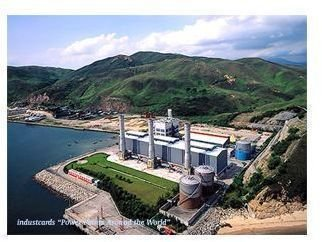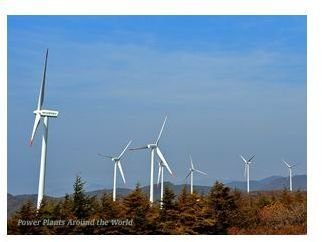# The need for Levelized Cost of Energy.

Energy conversion to electricity can be in many ways. From the most common coal fired plants to the renewables like solar and wind, the methods are many. The investment pattern and the return on investment for each method is different making it difficult to compare cost and evaluate investment strategies.

The initial investment required to construct a coal fired power plant is comparatively less, about 1000 \$/kW. But the operational cost, mainly the cost of fuel, is very high which continues during the life of the plant, say twenty or thirty years. Also the fuel cost will be subject to escalation. The same is the case with a gas turbine power plant. The fuel prices are subject to high escalation costs.

On the other hand a nuclear power plant requires a very high investment, in the range of 3000 \$/kW. But in the long run the fuel costs are considerably less.

In the case of a solar power plant the initial investments are high, in terms of land area and equipment. The output energy units is very low only 12 to 20 % of the installed capacity, even though the input energy cost is zero. Wind, hydro and geothermal power plants also require high initial investment, but considerably lower operational costs. Also many renewable energy projects get government incentives.

Today carbon capture costs are also a consideration for investment analysis.These are some of the very different scenarios in power generation investment. How to compare and calculate the economics of each type of power generation? How to arrive at a cost to charge the consumer? The Levelized Cost of Energy (LCOE) is the method that is commonly used. The method can also compare different technologies competing for a utility bid. Utilities use this to calculate the electricity cost to be charged to the consumer. In the electricity bidding market this LCOE is a very important factor.

## What is Levelized Cost of Energy?

Very simply put this is the sum of all the costs incurred during the lifetime of the project divided by the units of energy produced during the lifetime of the project, expressed as \$/kwhr.

Since most of the costs or expenses and the sales revenue occur in a future time, one has to account for the time value of money and the risks. This is done by calculating the Present Value of these cash flows.

A discount rate is used to calculate the Present Value. The discount rate varies within organizations and industries. This takes into account the risk factors, economic fundamentals, the investment mix, and the debt structuring.

The present value of the future returns less the investment in the beginning gives the Net Present Value (NPV). The \$/Kwhr rate used to calculate sales revenue to achieve a zero NPV is the Levelized Cost of Energy.

## Calculation of LCOE

To calculate LCOE one would require

• The capacity of the plant expressed in megawatts or kilowatts. The capacity can remain the same throughout the lifetime or can be reduced due to operational wear and tear.

• The yearly plant load factor . This accounts for the availability and utilization of the plant, the maintenance outages, and load reductions due to demand or machine defects.

This two will give the units of electricity generated during the life time of the plant. This together with unit cost of electricity will give the Sales revenue.

The costs can be of five major types:

• Investment costs. This includes the yearly interest charges, the paybacks, and other financial charges. The initial investment or the overnight charges is considered as occurring at the end of the construction phase of the project and is used to calculate the Net Present Value.

• Taxes. Depending on regulations and policies. Also to be included are subsidies or incentives for renewable power.

• Fuel cost. To calculate this one would require the heat rate or efficiency of the plant (kilojoules per kilo watt hour) , the cost of fuel (\$/kg or \$/Kg) and escalations to the cost of the fuel.

• Operation and maintenance costs. This is taken as a fixed number for each unit of electricity generated.

• Depreciation and major replacement costs.To calculate the Net Present Value one will require the discount rate expressed as percentage. It could be in the range of 10 to 18 %, which is matter of national and organizational policies.

Using an Excel spreadsheet will be the easiest way to do this calculation.

The cost can be calculated for each year. The sales revenue for each year is calculated based on the units generated and an assumed value of unit electricity cost.

The difference of the sales revenue and the costs give the yearly cash flow. The present value for this series of cash flow is calculated by the NPV function in Excel. The present value less the initial investment gives the Net Present Value.

The assumed value of electricity cost is adjusted or iterated to get a zero NPV. The Goal seek function in an Excel spreadsheet also can be used for this.

The unit electricity cost to achieve zero NPV is the Levelized Cost of Energy.